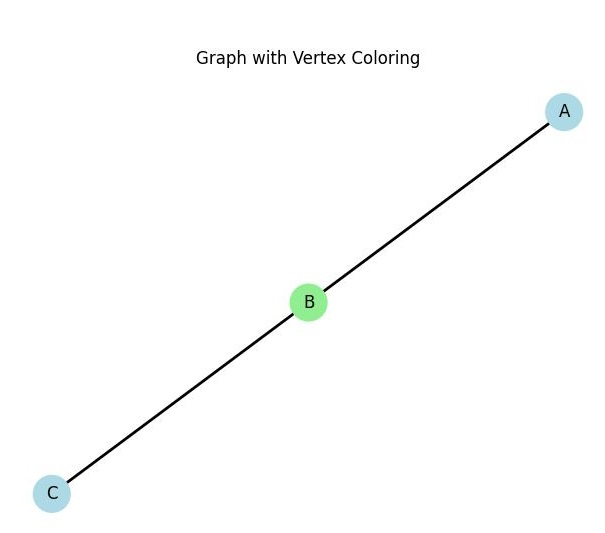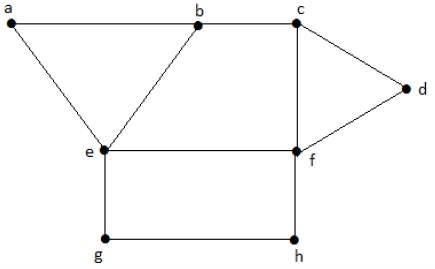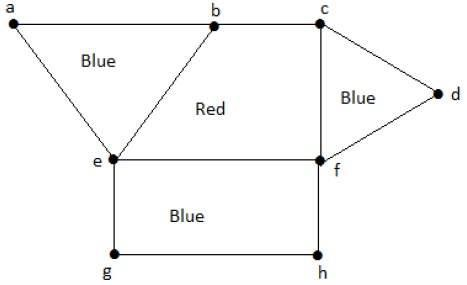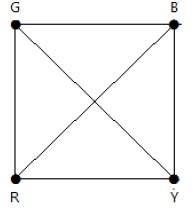# Graph Theory - Coloring

Graph coloring is nothing but a simple way of labelling graph components such as vertices, edges, and regions under some constraints. In a graph, no two adjacent vertices, adjacent edges, or adjacent regions are colored with minimum number of colors. This number is called the chromatic number and the graph is called a properly colored graph.

While graph coloring, the constraints that are set on the graph are colors, order of coloring, the way of assigning color, etc. A coloring is given to a vertex or a particular region. Thus, the vertices or regions having same colors form independent sets.

## Vertex Coloring

Vertex coloring is an assignment of colors to the vertices of a graph ‘G’ such that no two adjacent vertices have the same color. Simply put, no two vertices of an edge should be of the same color.

### Chromatic Number

The minimum number of colors required for vertex coloring of graph ‘G’ is called as the chromatic number of G, denoted by X(G).

χ(G) = 1 if and only if 'G' is a null graph. If 'G' is not a null graph, then χ(G) ≥ 2.

ExampleNote − A graph ‘G’ is said to be n-coverable if there is a vertex coloring that uses at most n colors, i.e., X(G) ≤ n.

## Region Coloring

Region coloring is an assignment of colors to the regions of a planar graph such that no two adjacent regions have the same color. Two regions are said to be adjacent if they have a common edge.

Example

Take a look at the following graph. The regions ‘aeb’ and ‘befc’ are adjacent, as there is a common edge ‘be’ between those two regions.Similarly, the other regions are also coloured based on the adjacency. This graph is coloured as follows −Example

The chromatic number of Kn is

• n
• n–1
• [n/2]
• [n/2]

Consider this example with K4.In the complete graph, each vertex is adjacent to remaining (n – 1) vertices. Hence, each vertex requires a new color. Hence the chromatic number of Kn = n.

## Applications of Graph Coloring

Graph coloring is one of the most important concepts in graph theory. It is used in many real-time applications of computer science such as −

• Clustering
• Data mining
• Image capturing
• Image segmentation
• Networking
• Resource allocation
• Processes scheduling